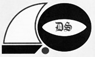# Math Exam (Grade 2 C)

Approved & Edited by ProProfs Editorial Team
The editorial team at ProProfs Quizzes consists of a select group of subject experts, trivia writers, and quiz masters who have authored over 10,000 quizzes taken by more than 100 million users. This team includes our in-house seasoned quiz moderators and subject matter experts. Our editorial experts, spread across the world, are rigorously trained using our comprehensive guidelines to ensure that you receive the highest quality quizzes.
| By Laragh
L
Laragh
Community Contributor
Quizzes Created: 2 | Total Attempts: 326
Questions: 15 | Attempts: 207Settings• 1.

### 6 x 5 =

• A.

30

• B.

36

• C.

40

• D.

25

A. 30
Explanation
The correct answer is 30 because when you multiply 6 by 5, you get the product of 30.

Rate this question:

• 2.

### The sum of 150+ 20 =

170
Explanation
The given question asks for the sum of 150 and 20. To find the sum, we add the two numbers together. So, adding 150 and 20 gives us a total of 170.

Rate this question:

• 3.

### The difference of 600 - 200 =

400
Explanation
The given question asks for the difference between 600 and 200. To find the difference, we subtract the smaller number (200) from the larger number (600). Therefore, the difference between 600 and 200 is 400.

Rate this question:

• 4.

### The sum of 600 + 200 =

800
Explanation
The question asks for the sum of 600 and 200. To find the sum, we add the two numbers together. Therefore, the sum of 600 + 200 is 800.

Rate this question:

• 5.

### The sum of 300 + 200 =

500
Explanation
The sum of 300 and 200 is 500 because when you add 300 and 200 together, you get a total of 500.

Rate this question:

• 6.

### The difference of 169 - 9 =

160
Explanation
The difference between 169 and 9 is obtained by subtracting 9 from 169, which equals 160.

Rate this question:

• 7.

### The difference of 153- 53 =

100
Explanation
The given question asks for the difference between 153 and 53. By subtracting 53 from 153, we get 100. Therefore, the correct answer is 100.

Rate this question:

• 8.

### 100 + 20 + 7 < 172

• A.

True

• B.

False

A. True
Explanation
The given inequality states that 100 + 20 + 7 is less than 172. By performing the addition, we get 127, which is indeed less than 172. Therefore, the answer is true.

Rate this question:

• 9.

### 300 + 40 + 5 < 354

• A.

True

• B.

False

A. True
Explanation
The inequality 300 + 40 + 5 < 354 is true because when we add the numbers on the left side, we get 345 which is indeed less than 354. Therefore, the answer is true.

Rate this question:

• 10.

### 300 + 30 + 6 = 336

• A.

True

• B.

False

A. True
Explanation
The given equation states that 300 + 30 + 6 equals 336. Upon calculation, we can verify that this statement is indeed true.

Rate this question:

• 11.

### 100 + 10 + 3 > 130

• A.

True

• B.

False

B. False
Explanation
The given expression is 100 + 10 + 3 > 130. When we simplify this expression, we get 113 > 130. However, this statement is false because 113 is not greater than 130. Therefore, the correct answer is False.

Rate this question:

• 12.

### 200 + 40 + 8 < 148

• A.

True

• B.

False

B. False
Explanation
The given inequality states that 200 + 40 + 8 is less than 148. However, this statement is not true because 200 + 40 + 8 equals 248, which is greater than 148. Therefore, the correct answer is false.

Rate this question:

• 13.

### 4 x 7 =

• A.

28

• B.

38

• C.

32

• D.

27

A. 28
Explanation
The correct answer is 28 because when you multiply 4 by 7, you are essentially adding 4 seven times. So, 4 + 4 + 4 + 4 + 4 + 4 + 4 equals 28.

Rate this question:

• 14.

### 2 x 8 =

• A.

24

• B.

18

• C.

16

• D.

10

C. 16
Explanation
The correct answer is 16 because when you multiply 2 by 8, you get the product of 16.

Rate this question:

• 15.

### 8 x 7 =

• A.

65

• B.

56

• C.

63

• D.

72

B. 56
Explanation
The correct answer is 56 because when you multiply 8 by 7, you get the product of 56.

Rate this question:

Related TopicsBack to top